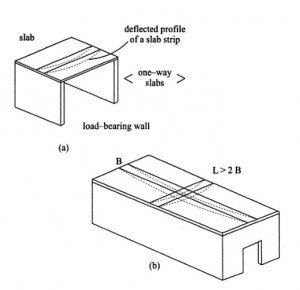# Design Procedure Steps for One Way Slab | Slab Design Steps

## Design Procedure for One Way Slab

Here are the steps for the Design Procedure for One Way Slab in steps.For those who do not know what are the design requirements of a slab.Before going to this topic, Please read it.

There are two types of slab classification based on the direction of bending occurs in these slabs.The two classifications are one-way slab and two-way slab.The basic difference between these slabs are one-way slab bends only in one direction and two-way slab bends in two directions as shown in the below figure.Let’s check what are the Design Procedure Steps for One Way Slab in the below section.

## Design of One way slab

1.Based on the stiffness requirement assume suitable depth and calculate the effective span2.Calculate the loads acting on the slab.

3.Calculate factored moment and shear force by considering the one-meter width of the slab

For simply supported slab

Factored moment =Mu= Wu× l²/ 8

Shear Force = Vu = Wu×l / 2

4.Determine the minimum depth required to resist bending moment by equating

Mu= Mulim = k×fck×bd²

b=1000mm

k=0.138 For Fe 415 steel and  k=0.148 for mild steel

The depth provided should be more than this value

5.Determine the area of steel considering meter width of the slab.

6.Determine the spacing of bars by using

S= ast/Ast ×1000

ast – area of bar used

Ast- total area of steel required

Spacing should be more than 3d or 300mm whichever is less

7.Distribution steel provides minimum reinforcement as distribution steel at a spacing of 5d or 450mm whichever is less.

8.Slab is checked for deflection shear and development length.In case of slabs( thin members), no need of even minimum shear reinforcement.Shear due to loads always must be less than the shear strength of concrete.

The below video shows the design of one-way slab